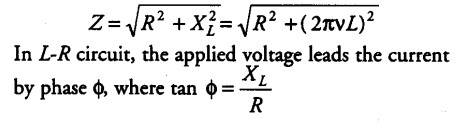# The graphs (a) and (b) represent the variation of the opposition

(i) The graphs (a) and (b) represent the variation of the opposition offered by the circuit element to the flow of alternating current with frequency of the applied emf. Identify the circuit elements corresponding to each graph.(ii) Write the expression for the impedance offered by the series combination of the above two elements connected across the AC source. Which will be ahead in phase in this circuit, voltage or current?

(i) From graph (a), it is clear that resistance (opposition to current) is not changing with frequency, i.e. resistance does not depend on frequency of applied voltage, so the circuit element here is pure resistive ®. From graph (b), it is clear that resistance increases linearly with frequency, so the circuit element here is inductive in nature.
(ii) Impedance offered by the series of resistance and inductor